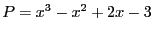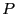Next: Sturm method Up: Budan-Fourier method Previous: Implementation   Contents

Example

Let, In be the interval [0,2] and the procedure:

Coeff(1)= -3;Coeff(2)=2;Coeff(3)=-1;Coeff(4)=1;
P=INTERVAL(0.0,2.0);
Num= Budan_Fourier_Interval(3,Coeff,P);
Num is 3 meaning thathas either 3 or 1 roots in the interval. Now assume that we have an interval polynomial:

Coeff_App(1)= INTERVAL(-3.1,-2.9);
Coeff_App(2)=INTERVAL(1.9,2.1);
Coeff_App(3)=INTERVAL(-1.1,-0.9);
Coeff_App(4)=INTERVAL(0.9,1.1);
Num= Budan_Fourier_Interval(Degree,Coeff_App,P,&Confidence);
Num is also 3 with Confidence=1 meaning that the number of roots for any polynomial is either 3 or 1.

Jean-Pierre Merlet 2012-12-20